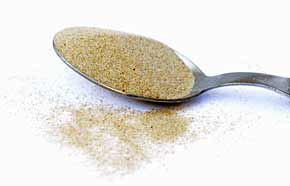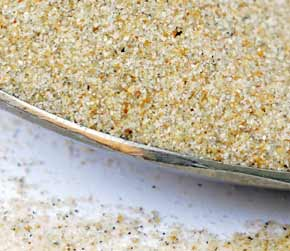﻿ Beach Sand 1 cubic meter volume to cups US converter

beach sand conversion

Amount: 1 cubic meter (m3) of volume Equals: 4,226.75 cups US (cup us) in volume

Converting cubic meter to cups US value in the beach sand units scale.

TOGGLE :   from cups US into cubic meters in the other way around.

beach sand from cubic meter to cup US Conversion Results:

Enter a New cubic meter Amount of beach sand to Convert From

* Whole numbers, decimals or fractions (ie: 6, 5.33, 17 3/8)
* Precision is how many numbers after decimal point (1 - 9)

Enter Amount :
Decimal Precision :

CONVERT :   between other beach sand measuring units - complete list.

Conversion calculator for webmasters.

Beach sand weight vs. volume units

Beach sand has quite high density, it's heavy and it easily leaks into even tiny gaps or other opened spaces. No wonder it absorbs and conducts heat energy from the sun so well. However, this sand does not have the heat conductivity as high as glass does, or fireclay and firebricks, or dense concrete. A fine beach sand in dry form was used for taking these measurements.Convert beach sand measuring units between cubic meter (m3) and cups US (cup us) but in the other reverse direction from cups US into cubic meters.

 conversion result for beach sand: From Symbol Equals Result To Symbol 1 cubic meter m3 = 4,226.75 cups US cup us

Converter type: beach sand measurements

This online beach sand from m3 into cup us converter is a handy tool not just for certified or experienced professionals.

First unit: cubic meter (m3) is used for measuring volume.
Second: cup US (cup us) is unit of volume.

beach sand per 4,226.75 cup us is equivalent to 1 what?

The cups US amount 4,226.75 cup us converts into 1 m3, one cubic meter. It is the EQUAL beach sand volume value of 1 cubic meter but in the cups US volume unit alternative.

How to convert 2 cubic meters (m3) of beach sand into cups US (cup us)? Is there a calculation formula?

First divide the two units variables. Then multiply the result by 2 - for example:
4226.7528377304 * 2 (or divide it by / 0.5)

QUESTION:
1 m3 of beach sand = ? cup us

1 m3 = 4,226.75 cup us of beach sand

Other applications for beach sand units calculator ...

With the above mentioned two-units calculating service it provides, this beach sand converter proved to be useful also as an online tool for:
1. practicing cubic meters and cups US of beach sand ( m3 vs. cup us ) measuring values exchange.
2. beach sand amounts conversion factors - between numerous unit pairs variations.
3. working with mass density - how heavy is a volume of beach sand - values and properties.

International unit symbols for these two beach sand measurements are:

Abbreviation or prefix ( abbr. short brevis ), unit symbol, for cubic meter is:
m3
Abbreviation or prefix ( abbr. ) brevis - short unit symbol for cup US is:
cup us

One cubic meter of beach sand converted to cup US equals to 4,226.75 cup us

How many cups US of beach sand are in 1 cubic meter? The answer is: The change of 1 m3 ( cubic meter ) volume unit of beach sand measure equals = to volume 4,226.75 cup us ( cup US ) as the equivalent measure within the same beach sand substance type.

In principle with any measuring task, switched on professional people always ensure, and their success depends on, they get the most precise conversion results everywhere and every-time. Not only whenever possible, it's always so. Often having only a good idea ( or more ideas ) might not be perfect nor good enough solution. If there is an exact known measure in m3 - cubic meters for beach sand amount, the rule is that the cubic meter number gets converted into cup us - cups US or any other beach sand unit absolutely exactly.

Conversion for how many cups US ( cup us ) of beach sand are contained in a cubic meter ( 1 m3 ). Or, how much in cups US of beach sand is in 1 cubic meter? To link to this beach sand cubic meter to cups US online converter simply cut and paste the following.
The link to this tool will appear as: beach sand from cubic meter (m3) to cups US (cup us) conversion.

I've done my best to build this site for you- Please send feedback to let me know how you enjoyed visiting.